By Topic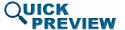• Abstract
• Authors
• Figures
• Multimedia
• References
• Cited By
• Keywords

# Dynamic spectral mask construction for radar transmission based on communication receiver locations

Dynamic spectrum allocation is becoming a means of allocating spectrum based on users and available frequencies in surrounding geographic regions. Presently, spectral masks are applied to radar transmission based on specifications from governmental regulatory agencies. This paper describes a concept for dynamic spectral mask determination based on the location and acceptable interference power levels at frequencies used by nearby communication receivers. This concept will be applied going forward to allow information from wireless networks about surrounding users to be applied in dynamically constraining radar transmitter spectra.

SECTION I

## INTRODUCTION

Sharing spectrum between radar and communications applications is becoming more difficult due to the prevalence of wireless broadband transmission and the consistent increase in users of the frequency spectrum. Clever nondisruptive approaches will need to be innovative to provide enough spectrum for these ever-growing applications, many of which are governmental and safety-related.

A new and growing approach for sharing spectrum is dynamic spectrum allocation, where frequency bands may be borrowed temporarily from primary users if they are not using their systems. An example of this is in the 5 GHz band, which is presently shared between radar and WiFi communications. To use this spectrum where radar is the primary user, the WiFi system must check for radar transmission before using the frequency band. If a radar is detected in the band at any time during use, the WiFi system must immediately vacate the spectrum.

The present paper describes the setup of how radar transmission spectral masks can be adapted based upon wireless handset users in the surrounding geographic region. This approach will allow circuit and waveform optimization algorithms developed by Fellows  and Eustice  to be applied based on surrounding spectrum users rather than on a traditional regulatory mask.

SECTION II

## SPECTRUM SHARING APPROACH OVERVIEW

Consider a radar transmitter located near multiple communication receivers. It is assumed that the radar transmitter is located at coordinates (0,0), and communication receiver handsets are located at positions that are known. Further, the maximum acceptable power density that can be tolerated by the receivers at their operating frequencies are assumed to be known to the transmitter. This information might be known either through a wireless network in which the coordinates and acceptable interference levels are communicated to the radar, or through radar detection itself and estimated values of harmful interference based on whatever knowledge of the receivers is available. We have constructed a simulation setup in which receiver locations and acceptable power density can be randomly generated using MATLAB.

The Friis transmission equation allows calculation of the power at a receiver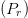$(P_{r})$ based on the transmitted power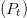$(P_{t})$, the distance from the transmitter to the receiver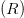$(R)$, and the antenna gains of both the transmitter and receiver antennas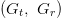$(G_{t},\ G_{r})$ :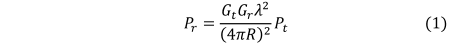TeX Source

To simplify the problem, isotropic radiation is assumed for both the transmitter and receiver antennas. In a real scenario, this is far from accurate. However, it can be seen that these scenarios can be easily placed into consideration using equation (1). For isotropic radiation,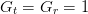$G_{t}=G_{r}=1$ in (1) to allow simple solution, based only upon the distance between the transmitter and receiver, the frequency of the receiver, and the acceptable interference power at the receiver. Thus we can write (1) as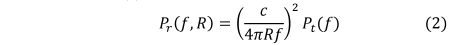TeX Source where we have set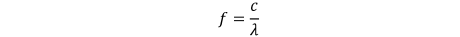TeX Source assuming free-space transmission. Assume there are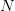$N$ handsets, the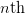$n\text{th}$ of which has parameters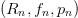$(R_{n},f_{n},p_{n})$ where

•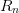$R_{n}$ is the distance from the transmitter to the$n\text{th}$ communication handset,

•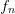$f_{n}$ is the frequency at which the handset operates, and

•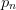$p_{n}$ is the maximum acceptable power per bandwidth to the$n\text{th}$ handset.

We will assume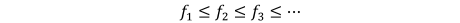TeX Source

Then, from (2), if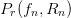$P_{r}(f_{n},R_{n})$ is the power received by the nth handset, then we requireTeX Source

Figure 1 shows the construction of a mask for a simple example using 4 handsets. In the case shown, the mask is placed a certain specified level below the maximum acceptable power.

Fig. 1. Spectral mask construction based on the transmitted power related to maximum acceptable power at the handsets and handset positions and operating frequencies.

To solve in dB for the transmitted power, the logarithm of (3) is computed: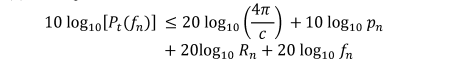TeX Source

So in terms of dB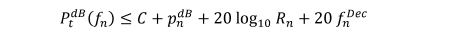TeX Source where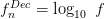$f_{n}^{Dec}=\log_{10} \ f$ measures frequency in decades and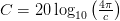$C=20\log_{10}\left(\frac{4\pi}{c}\right)$ is a constant.

SECTION III

## SPECIAL CASES

#### 1. Constant Range and Acceptable Handset Power

If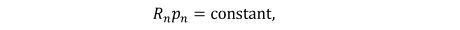TeX Source then the allowable transmitted power increases 20 dB per decade. This means that the allowable transmitted power to avoid interference with a given handset with fixed position and maximum acceptable interference power density (dBm/Hz) increases 20 dB per decade. As a result, when radar transmit power is held constant, interference is less likely at higher frequencies. However, for the same reason, the radar transmitter must transmit with a power that is higher by 20 dB per decade to illuminate a target at fixed distance, and with a power that is higher by 40 dB per decade to achieve the same power in the echo at the radar receiver. Thus, this tends to be a deceiving scenario: for radar operation it may actually be better to operate at lower frequencies in many cases.

#### 2. Worst Case

Rather than fitting to each individual communication handset's requirements, the mask can be piecewise fit to the worst case over given intervals if desired. This provides a smoother mask which is, in most cases, more restrictive on the radar transmission. Assume the parameters can be constrained by intervals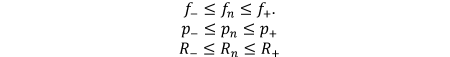TeX Source

Then at that distance, (3) becomes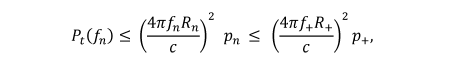TeX Source where, for each parameter, we have chosen the upper limit of the allowable interval. This corresponds to a rectangular mask with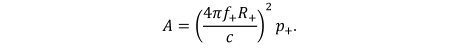TeX Source

Note the closer the handset is to the transmitter, the lower the mask amplitude. This rectangular mask is shown in Fig. 2.

Fig. 2. Rectangular “worst case” mask over a frequency interval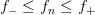$f_{-}\leq f_{n}\leq f_{+}$.

#### 3. Cell Specific

Equation (3) is satisfied when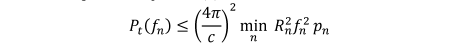TeX Source which gives us the rectangular mask with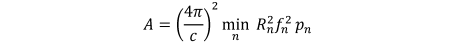TeX Source

A single worst case handset determines the mask amplitude.

#### 4. Piecewise Constant Sub Band Approach

Divide the band over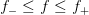$f_{-}\leq f\leq f_{+}$ into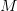$M$ sub bands with interval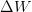$\Delta W$. The first band would be from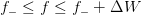$f_{-}\leq f\leq f_{-}+\Delta W$. The mth band is over the interval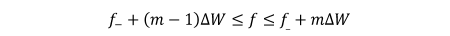TeX Source

Over the$m$th band, we require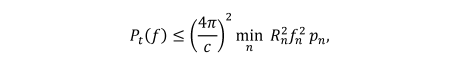TeX Source where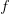$f$ and$f_{n}$ are in the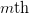$m\text{th}$ frequency band. Figure 3 shows the spectral mask construction for the piecewise constant sub-band approach.

Fig. 3. Spectral mask for the piecewise constant sub-band approach

#### 5. Piecewise Constant Approach

First, from (3), evaluate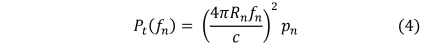TeX Source for all$n$. Run a fixed length min sliding window over the series to get the mask. Figure 4 shows an example of this approach.

Fig. 4. Plot of piecewise constant approach to constructing a spectral mask based on a sliding window scale. The blue line represents the connected maximum acceptable transmit power density and the red line represents the sliding-window spectral mask.
SECTION IV

## SIMULATION RESULTS

A simulation setup has been created in MATLAB to allow the development of this tool, which we plan to develop with increasing complexity. Use of this developing tool is briefly demonstrated using two simple scenarios. The frequency band from 3400 to 3700 MHz is used for illustration purposes, as this is a band that is presently used (at least in part) for spectrum sharing between radar and communications. In fact, much of the S-band radar allocation has been re-allocated for radar and communications sharing. The handset acceptable power density (dBm/Hz), frequency, and location can either be either randomly generated by the program or input on a spreadsheet to the program.

### A. Simulation A

Figure 5 shows a simple scenario in which the radar transmitter, notated in red, is located at the coordinates (0,0) and handset receivers are located at the positions marked by ‘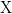$\text{X}$’ on the diagram. 10 handsets are used, all with frequencies between 3400 and 3700 MHz (this frequency range represents an actual frequency range where radar and communications spectrum sharing is in use). The handset settings are generated using the following inputs to the random generator used in MATLAB:

• Maximum receiver acceptable power density range: −75 to −65 dBm/Hz

• Radar transmitter power density: 20 dBm/Hz

• Frequency range: 3400 to 3700 MHz

• X location: −50 to 50 meters

• Y location: −50 to 50 meters

• Number of devices: 10

Based on the frequencies and locations of the handset receivers, the receiver acceptable power density, and the radar transmitter power density needed for the radar application, the spectral mask can be dynamically constructed. Figure 6 shows the spectral mask for the scenario in Fig. 5. The algorithm chooses the main transmission band as 3.506 GHz to 3.573 GHz (bandwidth of 66.725 MHz), because this is the largest gap between communication receiver handset frequencies. The main transmission band part of the mask is indicated in red in Fig. 6. The remaining part of the mask, constructed based on handset data, is shown in blue.

The spectral mask is based on the power density of both the in-band transmission of the radar and the maximum acceptable power density transmitted at the frequencies of the handsets (traced to the transmitter using equation (2)).

Fig. 5. Simulation A scenario with radar transmitter and communication receiver locations. The radar is notated in red and the handsets are in blue.
Fig. 6. Simulation A spectral mask for radar transmission based on acceptable power density levels at each communication receiver. The spectral mask has a bandwidth of 66.725 MHz, with a low frequency of 3.506 GHz and a high frequency of 3.573 GHz.

### B. Simulation B

A second simulation was performed using the following settings. A notable change in this simulation is the much larger number of devices: 1500 communication receiver handsets are used, all with operating frequencies between 3400 and 3700 MHz.

• Maximum receiver acceptable power density range: −85 to −65 dBm/Hz

• Radar transmitter power density: 20 dBm/Hz

• Frequency range: 3400 to 3700 MHz

• X location: −50 to 50 meters

• Y location: −50 to 50 meters

• Number of devices: 1500

The locations of the communication handsets are shown in Fig. 7, assuming that the radar transmitter, again notated in red, is located at the coordinates (0,0). This is a very busy environment. In Fig. 8, the dynamic spectral mask is plotted by connecting the maximum transmit power density at the handset frequencies to the in-band transmission power. Again, the algorithm selects the widest frequency range available in the pre-specified range to perform the transmission. Because of the significantly higher number of devices, it can be seen that the bandwidth for transmission is 1.312 MHz, significantly smaller than in Simulation A. This will force the radar transmitter to adjust to fit a tighter spectral mask. The difference between Simulations A and B represents a scenario in which a radar transmitter must adapt from a less crowded to more crowded wireless environment.

Fig. 7. Simulation B scenario with radar transmitter (red) and communication receiver locations (blue).
Fig. 8. Simulation B spectral mask, zoomed in for clarity, for radar transmission based on acceptable power density levels at each communication receiver. The spectral mask has a bandwidth of 1.312 MHz, with a low frequency of 3.543 GHz and a high frequency of 3.565 GHz.
SECTION V

## CONCLUSIONS

An initial simulation has been presented for the creation of a dynamic spectral mask for radar transmission based on the locations and maximum acceptable power density levels of surrounding communication receiver handsets, which would ensure that a wireless communication receiver does not become victimized by the radar transmission. This work represents a first step toward a more complex platform in which the spectral mask for radar transmission is based on its surroundings and on system specifications. This platform can be expanded upon by considering directivity of the radar transmitter and communication receiver handsets, and other complicating factors such as atmospheric attenuation and radar transmitter rotation.

Next steps to expanding this work include using the dynamic spectral mask to control load impedance  and waveform optimizations . Putting this framework in place explores the upper-level scenario control of the algorithms that will provide for reconfigurable radar transmitters to optimize their circuitry and waveform to meet spectral output requirements while achieving desired range/Doppler resolution requirements (based on the ambiguity function) and power-added efficiency.

### Acknowledgment

This work has been funded by the National Science Foundation (Grant No. ECCS-1343316).

## Footnotes

No Data Available

None

## Keywords

### Authors Keywords

cognitive radar, interchannel interference, power amplifiers, spectral mask

## Corrections

None

This paper appears in:
2016 Texas Symposium on Wireless and Microwave Circuits and Systems (WMCS)
Conference Date(s):
March 31 2016-April 1 2016
Conference Location:
Waco, TX
On page(s):
1 - 5
E-ISBN:
No Data Available
Print ISBN:
New-2005_Electronic_978-1-5090-2755-2
INSPEC Accession Number:
16341410
Digital Object Identifier:
10.1109/WMCaS.2016.7577484
Date of Current Version:
2016-09-29
Date of Original Publication:
No Data Available